# Scatter plot in Plotly using graph_objects class

• Last Updated : 30 Aug, 2021

Plotly is a Python library which is used to design graphs, especially interactive graphs. It can plot various graphs and charts like histogram, barplot, boxplot, spreadplot and many more. It is mainly used in data analysis as well as financial analysis. plotly is an interactive visualization library.

## Scatter plot using graph_objects class

Scatter plot are those charts in which data points are represented horizontally and on vertical axis to show that how one variable affect on another variable. The scatter() method of graph_objects class produces a scatter trace. The mode of the property decides the appearance of data points.

Attention geek! Strengthen your foundations with the Python Programming Foundation Course and learn the basics.

To begin with, your interview preparations Enhance your Data Structures concepts with the Python DS Course. And to begin with your Machine Learning Journey, join the Machine Learning - Basic Level Course

Syntax: plotly.graph_objects.Scatter(arg=None, cliponaxis=None, connectgaps=None, customdata=None, customdatasrc=None, dx=None, dy=None, error_x=None, error_y=None, fill=None, fillcolor=None, groupnorm=None, hoverinfo=None, hoverinfosrc=None, hoverlabel=None, hoveron=None, hovertemplate=None, hovertemplatesrc=None, hovertext=None, hovertextsrc=None, ids=None, idssrc=None, legendgroup=None, line=None, marker=None, meta=None, metasrc=None, mode=None, name=None, opacity=None, orientation=None, r=None, rsrc=None, selected=None, selectedpoints=None, showlegend=None, stackgaps=None, stackgroup=None, stream=None, t=None, text=None, textfont=None, textposition=None, textpositionsrc=None, textsrc=None, texttemplate=None, texttemplatesrc=None, tsrc=None, uid=None, uirevision=None, unselected=None, visible=None, x=None, x0=None, xaxis=None, xcalendar=None, xsrc=None, y=None, y0=None, yaxis=None, ycalendar=None, ysrc=None, **kwargs)

Parameters:

Example:

## Python3

 `import` `plotly.graph_objects as px``import` `numpy as np`  `# creating random data through randomint``# function of numpy.random``np.random.seed(``42``)` `random_x``=` `np.random.randint(``1``,``101``,``100``)``random_y``=` `np.random.randint(``1``,``101``,``100``)` `plot ``=` `px.Figure(data``=``[px.Scatter(``    ``x ``=` `random_x,``    ``y ``=` `random_y,``    ``mode ``=` `'markers'``,)``])``                 ` `plot.show()`

Output: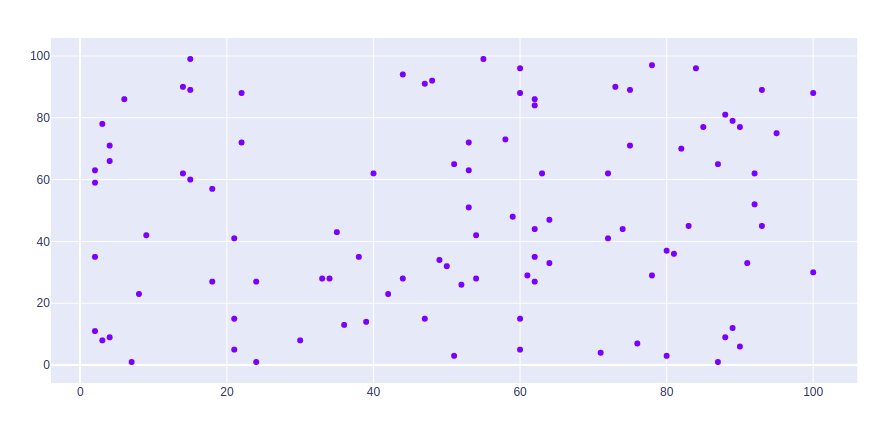## Presenting Scatter with a Color Dimension

Color scale can be shown using the showscale parameter. This parameter takes a boolean value. If the value is true then the scale is shown otherwise not.

Example:

## Python3

 `import` `plotly.graph_objects as go``import` `numpy as np`` ` `n ``=` `10000``plot ``=` `go.Figure(data``=``[go.Scatter(``    ``x ``=` `np.random.randn(n),``    ``mode ``=` `'markers'``,``    ``marker``=``dict``(``        ``color``=``np.random.randn(n),``        ``colorscale``=``'Viridis'``,``        ``showscale``=``True``    ``)``)``])``                 ` `plot.show()`

Output: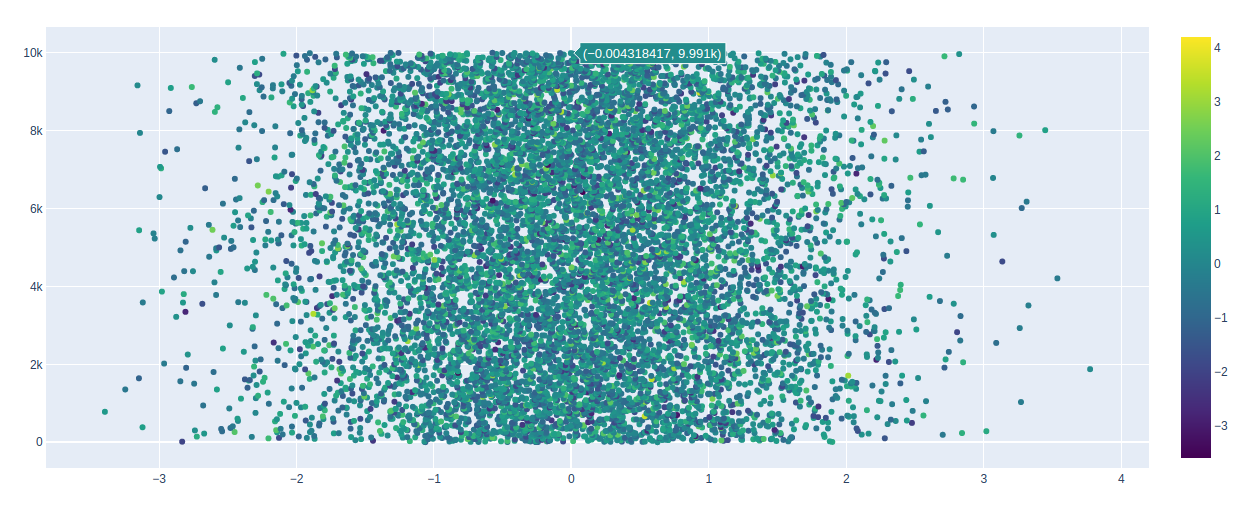## Styling Scatter Plots

In scatter plot can be styled using keywords arguments, let’s see the examples given below:

Example 1: Changing the color of the graph

## Python3

 `import` `plotly.graph_objects as px``import` `numpy as np`  `# creating random data through randomint``# function of numpy.random``np.random.seed(``42``)` `random_x``=` `np.random.randint(``1``,``101``,``100``)``random_y``=` `np.random.randint(``1``,``101``,``100``)` `plot ``=` `px.Figure(data``=``[px.Scatter(``    ``x ``=` `random_x,``    ``y ``=` `random_y,``    ``mode ``=` `'markers'``,``    ``marker_color``=``'rgba(199, 10, 165, .9)'``)``])``                 ` `plot.show()`

Output: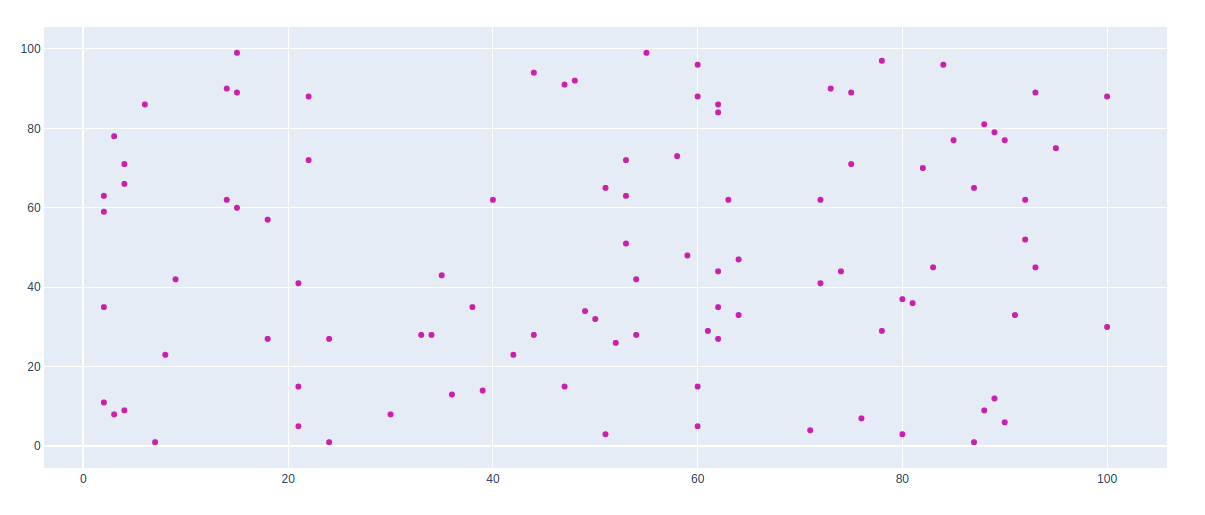Example 2: Using tips dataset

## Python3

 `import` `plotly.graph_objects as px``import` `plotly.express as go``import` `numpy as np` `df ``=` `go.data.tips()` `x ``=` `df[``'total_bill'``]``y ``=` `df[``'day'``]` `plot ``=` `px.Figure(data``=``[px.Scatter(``    ``x ``=` `x,``    ``y ``=` `y,``    ``mode ``=` `'markers'``,``    ``marker_color``=``'rgba(199, 10, 165, .9)'``)``])``           ` `plot.update_traces(mode``=``'markers'``, marker_size``=``10``)``      ` `plot.show()`

Output: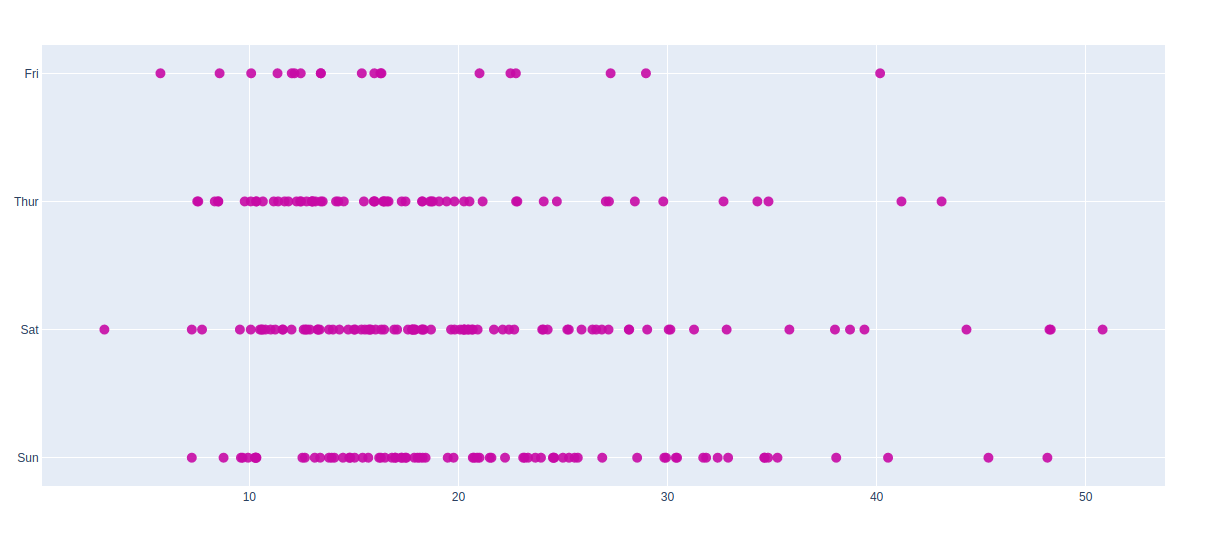## Bubble Scatter Plots

The bubble scatter plot can be created using the marker size. Marker size and color are used to control the overall size of the marker. Marker size helps to maintain the color inside the bubble in the graph.

Example:

## Python3

 `import` `plotly.graph_objects as px``import` `numpy as np`` `  `# creating random data through randomint``# function of numpy.random``np.random.seed(``42``)`` ` `random_x``=` `np.random.randint(``1``,``101``,``100``)``random_y``=` `np.random.randint(``1``,``101``,``100``)`` ` `plot ``=` `px.Figure(data``=``[px.Scatter(``    ``x ``=` `random_x,``    ``y ``=` `random_y,``    ``mode ``=` `'markers'``,``    ``marker_size ``=` `[``115``, ``20``, ``30``])``])``                  ` `plot.show()`

Output: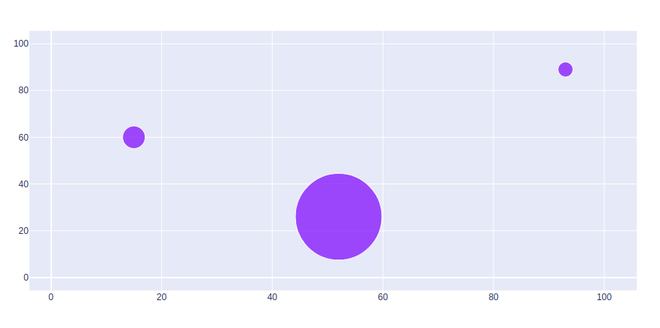My Personal Notes arrow_drop_up